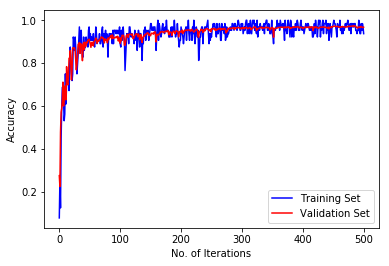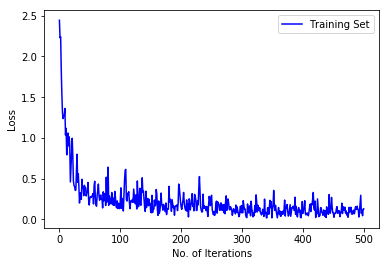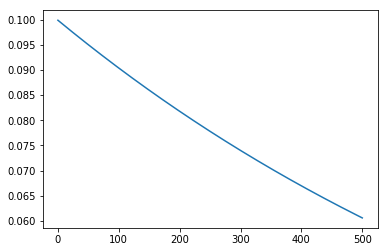# Mnist using caffe2 with Specific GPU use¶

##### Importing general packages¶
In :
import numpy as np
import cPickle
import csv
from matplotlib import pyplot as plt
%matplotlib inline

##### Importing Caffe2 Packages¶
In :
from caffe2.python import core,workspace,model_helper,brew,optimizer
from caffe2.proto import caffe2_pb2

##### Beaming Up Protocol¶

The requiered protocol files may be downloaded from https://www.kaggle.com/c/digit-recognizer/data

In :
raw_train = np.loadtxt('../Data/mnist_train.csv',delimiter=',')

##### Preprocessing Protocol¶

Let's create a 80/20 split of the provided training data to generate a validation set.
The new dataset structure can be seen in the output.

In :
train,val = np.split(raw_train,[int(0.8*raw_train.shape)])
print train.shape,val.shape

(48000, 785) (12000, 785)

##### Defining LeNet Architecture¶

Since we wish to run the experiments on the GPU lets specify the device option.

In :
device_option = caffe2_pb2.DeviceOption(device_type=caffe2_pb2.CUDA)


Now, lets define the LeNet architecture
Note: in caffe2 currently each layer requiers inputs dimension size too

In :
def AddLeNetModel(model):
with core.DeviceScope(device_option):
conv1 = brew.conv(model,'data', 'conv1', 1, 20, 5)
pool1 = brew.max_pool(model, conv1, 'pool1', kernel=2, stride=2)
conv2 = brew.conv(model, pool1, 'conv2', 20, 50, 5)
pool2 = brew.max_pool(model, conv2, 'pool2', kernel=2, stride=2)
fc3 = brew.fc(model, pool2, 'fc3', 50 * 4 * 4, 500)
fc3 = brew.relu(model, fc3, fc3)
pred = brew.fc(model, fc3, 'pred', 500, 10)
softmax = brew.softmax(model, pred, 'softmax')
return softmax

##### Calculating Accuracy¶
In :
def AddAccuracy(model, softmax):
accuracy = brew.accuracy(model, [softmax, 'label'], "accuracy")
return accuracy

##### Defining the Training Operators¶

Here we calculate the cross entropy loss and call the accuracy function to calculate the accuracy on the training set.
Most importantly we add the loss as a gradient in order to enable back propogation.
We also initialize the SGD solver along with the learning rate policy we follow.
Since, the learning rate policy is step and stepsize is 1 at every new iteration the learning rate would be

$$Base\ Learning\ Rate*\gamma^{iteration\ no - 1}$$
In :
def AddTrainingOperators(model, softmax):
# Loss Calculation
xent = model.LabelCrossEntropy([softmax, 'label'])
loss = model.AveragedLoss(xent, "loss")
# Calculating Accuracy
# Add loss to gradient for backpropogation
# Initializing the SGD the solver
opt = optimizer.build_sgd(model, base_learning_rate=0.1, policy="step", stepsize=1, gamma=0.999)


Let's define the batch size

In :
Batch_Size = 64

##### Lets Reset the Workspace¶
In :
workspace.ResetWorkspace()

Out:
True
##### Defining Training Model¶

Let's create a model that we will use for training.

In :
training_model = model_helper.ModelHelper(name="training_net")


Define the GPU ID to run experiment on

In :
gpu_no=0

##### To run on single GPU of choice¶
In :
training_model.net.RunAllOnGPU(gpu_id=gpu_no, use_cudnn=True)
training_model.param_init_net.RunAllOnGPU(gpu_id=gpu_no, use_cudnn=True)

##### Adding network and training operators to the training model¶
In :
soft=AddLeNetModel(training_model)

##### Initializing the network and loading it into the workspace¶
In :
workspace.RunNetOnce(training_model.param_init_net)
workspace.CreateNet(training_model.net,overwrite=True,input_blobs=['data','label'])

Out:
True
##### Saving snapshots¶

The following function saves layer weights for later use.
The weights are saved in a python dictionary with the keys as the blob name and value as the weights. Note, better ways exists at https://github.com/caffe2/caffe2/blob/master/caffe2/python/predictor/mobile_exporter.py

In :
Snapshot_location='snapshots/'
def save_snapshot(model,iter_no):
d={}
for blob in model.GetParams():
d[blob]=workspace.FetchBlob(blob)
cPickle.dump(d,open(Snapshot_location+str(iter_no),'w'))

##### Creating Validation Model¶

Lets create and define the validation model similar to the training model mentioned above.
Note, here we don't initialize the weights but use whatever are the existing values for the weights. We also don't add the training operators therefore there won't be any loss for the validation set.

In :
val_model = model_helper.ModelHelper(name="validation_net", init_params=False)
val_model.net.RunAllOnGPU(gpu_id=gpu_no, use_cudnn=True)
val_model.param_init_net.RunAllOnGPU(gpu_id=gpu_no, use_cudnn=True)
workspace.RunNetOnce(val_model.param_init_net)
workspace.CreateNet(val_model.net,overwrite=True,input_blobs=['data','label'])

Out:
True

Let's evaluate the performance of a given model on the entire validation set.
the function returns the averaged loss and accuracy

In :
def check_val():
accuracy = []
start=0
while start<val.shape:
l = val[start:start+Batch_Size,0].astype(np.int32)
batch = val[start:start+Batch_Size,1:].reshape(l.shape,28,28)
batch = batch[:,np.newaxis,...].astype(np.float32)
batch = batch*float(1./256)
workspace.FeedBlob("data", batch, device_option)
workspace.FeedBlob("label", l, device_option)
workspace.RunNet(val_model.net, num_iter=1)
accuracy.append(workspace.FetchBlob('accuracy'))
start+=l.shape
return np.mean(accuracy)


Let's define the interval at which we intend to take snapshots

In :
total_iterations = 501
Snapshot_interval=10
total_iterations = total_iterations * 64

In :
print workspace.Blobs()

[u'ONE_1_0', u'SgdOptimizer_0_lr_gpu0', u'accuracy', u'conv1', u'conv1_b', u'conv1_b_grad', u'conv1_grad', u'conv1_w', u'conv1_w_grad', u'conv2', u'conv2_b', u'conv2_b_grad', u'conv2_grad', u'conv2_w', u'conv2_w_grad', u'data', u'data_grad', u'fc3', u'fc3_b', u'fc3_b_grad', u'fc3_grad', u'fc3_w', u'fc3_w_grad', u'iteration_mutex', u'label', u'loss', u'loss_autogen_grad', u'optimizer_iteration', u'pool1', u'pool1_grad', u'pool2', u'pool2_grad', u'pred', u'pred_b', u'pred_b_grad', u'pred_grad', u'pred_w', u'pred_w_grad', u'softmax', u'softmax_grad', u'training_net/LabelCrossEntropy', u'training_net/LabelCrossEntropy_grad']

##### Running Training¶

We train the model on the training set and evaluate its performance on validation set after each iteration

In :
accuracy = []
val_accuracy = []
loss = []
lr = []
start=0
while start<total_iterations:
l = train[start:start+Batch_Size,0].astype(np.int32) # labels for a given batch
d=train[start:start+Batch_Size,1:].reshape(l.shape,28,28) # pixel values for each sample in the batch
d=d[:,np.newaxis,...].astype(np.float32)
d=d*float(1./256) # Scaling the pixel values for faster computation
workspace.FeedBlob("data", d, device_option)
workspace.FeedBlob("label", l, device_option)
workspace.RunNet(training_model.net, num_iter=1)
accuracy.append(workspace.FetchBlob('accuracy'))
loss.append(workspace.FetchBlob('loss'))
lr.append(workspace.FetchBlob('SgdOptimizer_0_lr_gpu0'))
#    lr.append(workspace.FetchBlob('conv1_b_lr'))
if start%Snapshot_interval == 0:
save_snapshot(training_model,start)
val_accuracy.append(check_val())
start+=Batch_Size


Lets evaluate the accuracy on the training and validation set for each iteration

In :
plt.plot(accuracy,'b',label='Training Set')
plt.plot(val_accuracy,'r',label='Validation Set')
plt.ylabel('Accuracy')
plt.xlabel('No. of Iterations')
plt.legend(loc=4)
plt.show()Lets evaluate the changes in loss values for each iteration of the training set

In :
plt.plot(loss,'b',label='Training Set')
plt.ylabel('Loss')
plt.xlabel('No. of Iterations')
plt.legend(loc=1)
plt.show()Note: Since for internal processing caffe2 negates the learning rate it currently returns negative of the actual learning rate, therefore we will inverse the signs before visualizing.

In :
lr = [-1*l for l in lr]
plt.plot(lr)
plt.show()##### Creating Testing Model¶

We create the test model, to predict results for the test set.
Note, that in this case we dont calculate the accuracy and in the input blobs we don't provide the labels

In :
testing_model = model_helper.ModelHelper(name="testing_net", init_params=False)
testing_model.net.RunAllOnGPU(gpu_id=gpu_no, use_cudnn=True)
testing_model.param_init_net.RunAllOnGPU(gpu_id=gpu_no, use_cudnn=True)
workspace.RunNetOnce(testing_model.param_init_net)
workspace.CreateNet(testing_model.net,overwrite=True,input_blobs=['data'])

Out:
True

Lets find the iteration that performs best on the validation set and for which we have a snapshot

In :
best = np.argmax(np.array(val_accuracy)[range(0,np.array(val_accuracy).shape,Snapshot_interval)])
best = best*Batch_Size*Snapshot_interval


Lets feed the best weight combination found above into the workspace

In :
params=cPickle.load(open(Snapshot_location+str(best),'rb'))
for blob in params.keys():
workspace.FeedBlob(blob,params[blob],device_option)


Let's predict the output for the test set

In :
#test=raw_train

In :
#results=[['ImageId','Label']]
results=[]
start=0
while start<test.shape:
raw_batch = test[start:start+Batch_Size,1:]
labels = test[start:start+Batch_Size,0]
batch = raw_batch.reshape(raw_batch.shape,28,28)
batch = batch[:,np.newaxis,...].astype(np.float32)
batch = batch*float(1./256)
workspace.FeedBlob("data", batch, device_option)
workspace.RunNet(testing_model.net, num_iter=1)
res = np.argmax(workspace.FetchBlob('softmax'),axis=1)
feat = workspace.FetchBlob('fc3')
#    for r in range(raw_batch.shape):
#        results.append([start+r+1,res[r]])
for r in range(raw_batch.shape):
temp=[]
for i,j in enumerate(feat[r].tolist()):
temp.append(str(i+1)+':'+str(j))
results.append([int(labels[r])]+temp)
start+=raw_batch.shape

##### Beaming Down Results¶
In :
with open('results.csv', "w") as output:
wr = csv.writer(output,delimiter=' ', lineterminator='\n')
wr.writerows(results)


Upload the csv file created above at https://www.kaggle.com/c/digit-recognizer/submit to evaluate the performance on the test set.
You should obtain an accuracy greater than 95% on the test set.

This completes the MNIST experiment

- Akshay Raj Dhamija